# 2023年成考高起点每日一练《数学(文史)》11月21日

## 试卷预览

• 1. 函数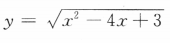的定义域是（）

A{x|-3≤x≤-1}

B{x|x≤-3或x≥-1}

C{x|1≤x≤3}

D{x|x≤1或x≥3}

• 2. 设集合S={(x,y)|xy>0},T={(x,y)|x>0,且y>0}，则

AS∪T=S

BS∪T=T

CS∩T=S

DS∩T=∅

• 3. b=0是直线y=kx+b过原点的（）

A充分但不必要条件

B必要但不充分条件

C充要条件

D既不充分也不必要条件

• 4. 函数y=2sinxcosx的最小正周期是（）

A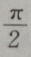B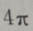C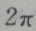D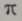• 1. 已知直线l的斜率为1，l过抛物线C：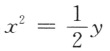的焦点，且与C交于A,B两点.
(I）求l与C的准线的交点坐标；
(II）求|AB|．
• 2. 在△ABC中，B=120°,C=30°,BC=4，求△ABC的面积．
• 3. 在△ABC中，AB=2，BC=3,B=60°，求AC及△ABC的面积
• 4. 设函数f(x)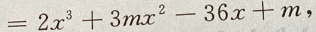且f'(-1)=-36 (Ⅰ)求m (Ⅱ)求f(x)的单调区间
• 1. 点（4,5)关于直线y=x的对称点的坐标为（）
• 2. 函数y=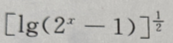的定义域是（）